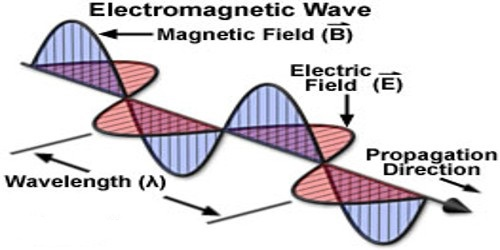# Characteristics of Electromagnetic Waves

Electromagnetic waves consist of oscillating electrical and magnetic fields, acting perpendicular to each other. Dissimilar ranges of electromagnetic waves can be classified according to their characteristics and how they interact with matter. Some examples include – radio waves, microwaves, x-rays, and visible light. These waves are transverse waves, similar to water waves in the ocean or the waves seen on a guitar string.

Characteristics of electromagnetic waves

Amplitude – The amplitude of these waves relates to its intensity or brightness. With visible light, the brightness is usually measured in lumens.

Wavelength – The wavelengths of these waves go from extremely long to extremely short and everything in between.

Velocity – The velocity of these waves in a vacuum is approximately 186,000 miles per second or 300,000 kilometers per second, the same as the speed of light.

Frequency – The frequency of any waveform equals the velocity divided by the wavelength. The units of measurement are in cycles per second or Hertz.

Other common Characteristics –

(i) Electromagnetic waves are produced by accelerated charges. So, accelerated charges are responsible to produce these waves.

(ii) They do not require any material medium for propagation. The inherent characteristic of an electromagnetic wave is its frequency. Their frequencies stay unmovable but its wavelength changes when the wave travels from one medium to another.

(iii) In an electromagnetic wave, the electric (E) and magnetic (B) field vectors are at right angles to each other and to the direction of propagation. Hence electromagnetic waves are transverse in nature.

(iv) Variation of maxima and minima in both E and B occur simultaneously. The refractive index of a material is given by: n = √μrϵr.

(v) They travel in vacuum or free space with a velocity 3 × 108 m s-1 given by the relation C = 1/√(μ0ε0)

0 – permeability of free space and ε0 – permittivity of free space)

(vi) The energy in an electromagnetic wave is equally divided between electric and magnetic field vectors. These waves are transverse in nature as they propagate by varying the electric and magnetic fields such that the two fields are perpendicular to each other.

(vii) The electromagnetic waves being chargeless, are not deflected by electric and magnetic fields. The energy is carried by the electric and magnetic fields of electromagnetic waves are equal, i.e. the electric energy (uE) and the magnetic energy (uM) are equal; uE = uM.

(viii) The light vector (also known as the electric vector) is the reason for the optical effects due to an electromagnetic wave.

(ix) In an electromagnetic wave, the oscillating electric and magnetic fields are in the same phase and their magnitudes have a constant ratio.

(x) There is a vector quantity S, called Poynting vector which represents the energy transferred by electromagnetic waves per second per unit area. S→ = 1μE→ × B→.